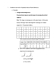# STATS 250 Lecture Notes - Lecture 8: Confidence Interval, Statistical Parameter, Standard Deviation

20 views2 pages
School
Department
Course
Professorazureswan549 and 37 others unlocked49
Verified Note
49 documents

## Document Summary

Chapter 5 part 2: learning about a population proportion. We cannot compute the true standard deviation of. Use sample data to estimate a population parameter. Standard error of the sample estimate gives an idea of how far away it tends to vary from the parameter value on average. Sample estimate (a few) standard deviations: the few standard deviations depends on how confident we want to be. We want to learn about a population proportion. We take a random sample and estimate p with a sample proportion. If the sample size is large, has a normal approximation: large: np 10 and n(1-p) 10, normal approximation: Each possible random samples provides a sample proportion value: a histogram of these possible values would like a normal distribution. A 95% confidence interval will include two standard deviations: replaced with an estimate (, this becomes a standard error, instead of a standard deviation. An approximate 95% confidence interval for the population proportion =

## Get access

\$8 USD/m\$10 USD/m
Billed \$96 USD annuallyHomework Help
Study Guides
Textbook Solutions
Class Notes
Textbook Notes
Booster Class
Class+
\$8 USD/m
Billed \$96 USD annuallyHomework Help
Study Guides
Textbook Solutions
Class Notes
Textbook Notes
Booster Class